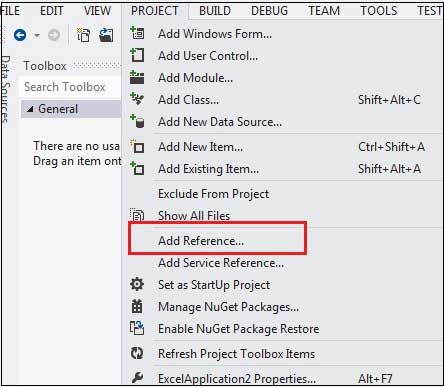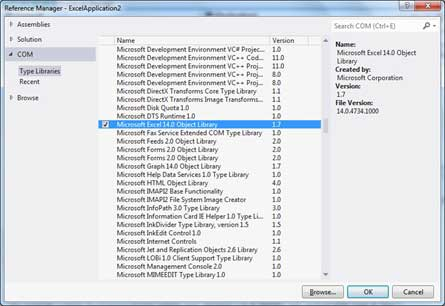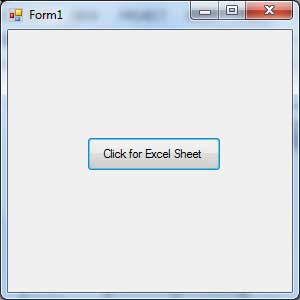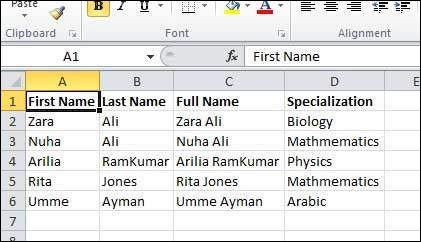# VB.Net - Excel Sheet

VB.Net provides support for interoperability between the COM object model of Microsoft Excel 2010 and your application.

To avail this interoperability in your application, you need to import the namespace Microsoft.Office.Interop.Excel in your Windows Form Application.

## Creating an Excel Application from VB.Net

Let's start with creating a Window Forms Application by following the following steps in Microsoft Visual Studio: File → New Project → Windows Forms Applications

Finally, select OK, Microsoft Visual Studio creates your project and displays following Form1.

Insert a Button control Button1 in the form.

Add a reference to Microsoft Excel Object Library to your project. To do this −• On the COM tab, locate Microsoft Excel Object Library and then click Select.• Click OK.

Double click the code window and populate the Click event of Button1, as shown below.

```'  Add the following code snippet on top of Form1.vb
Imports Excel = Microsoft.Office.Interop.Excel
Public Class Form1
Private Sub Button1_Click(sender As Object, e As EventArgs) Handles Button1.Click
Dim appXL As Excel.Application
Dim wbXl As Excel.Workbook
Dim shXL As Excel.Worksheet
Dim raXL As Excel.Range

' Start Excel and get Application object.
appXL = CreateObject("Excel.Application")
appXL.Visible = True

shXL = wbXl.ActiveSheet

shXL.Cells(1, 1).Value = "First Name"
shXL.Cells(1, 2).Value = "Last Name"
shXL.Cells(1, 3).Value = "Full Name"
shXL.Cells(1, 4).Value = "Specialization"

' Format A1:D1 as bold, vertical alignment = center.
With shXL.Range("A1", "D1")
.Font.Bold = True
.VerticalAlignment = Excel.XlVAlign.xlVAlignCenter
End With

' Create an array to set multiple values at once.
Dim students(5, 2) As String
students(0, 0) = "Zara"
students(0, 1) = "Ali"
students(1, 0) = "Nuha"
students(1, 1) = "Ali"
students(2, 0) = "Arilia"
students(2, 1) = "RamKumar"
students(3, 0) = "Rita"
students(3, 1) = "Jones"
students(4, 0) = "Umme"
students(4, 1) = "Ayman"

' Fill A2:B6 with an array of values (First and Last Names).
shXL.Range("A2", "B6").Value = students

' Fill C2:C6 with a relative formula (=A2 & " " & B2).
raXL = shXL.Range("C2", "C6")
raXL.Formula = "=A2 & "" "" & B2"

' Fill D2:D6 values.
With shXL
.Cells(2, 4).Value = "Biology"
.Cells(3, 4).Value = "Mathmematics"
.Cells(4, 4).Value = "Physics"
.Cells(5, 4).Value = "Mathmematics"
.Cells(6, 4).Value = "Arabic"
End With

' AutoFit columns A:D.
raXL = shXL.Range("A1", "D1")
raXL.EntireColumn.AutoFit()

' Make sure Excel is visible and give the user control
appXL.Visible = True
appXL.UserControl = True

' Release object references.
raXL = Nothing
shXL = Nothing
wbXl = Nothing
appXL.Quit()
appXL = Nothing
Exit Sub
Err_Handler:
MsgBox(Err.Description, vbCritical, "Error: " & Err.Number)
End Sub
End Class
```

When the above code is executed and run using Start button available at the Microsoft Visual Studio tool bar, it will show the following window −Clicking on the Button would display the following excel sheet. You will be asked to save the workbook.